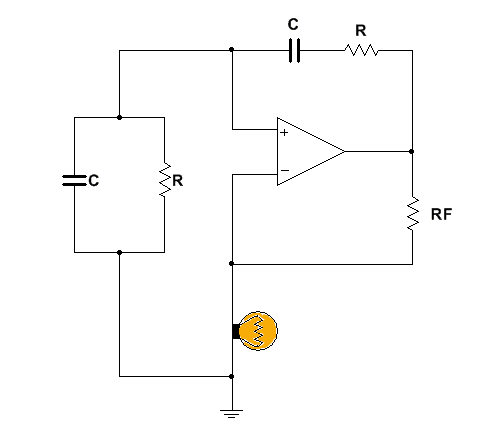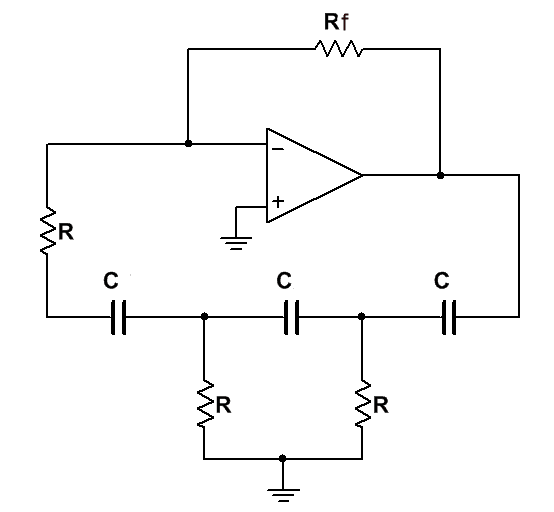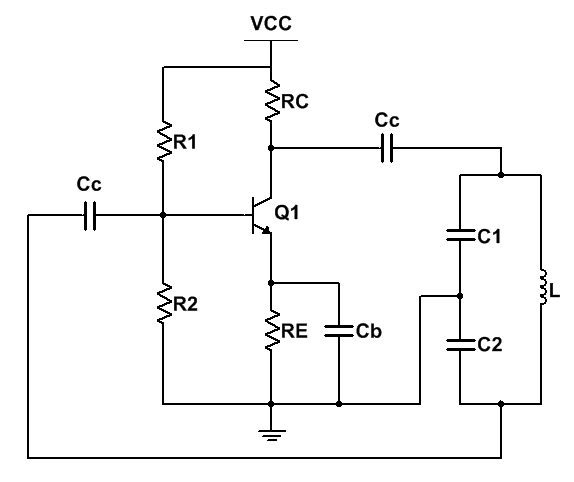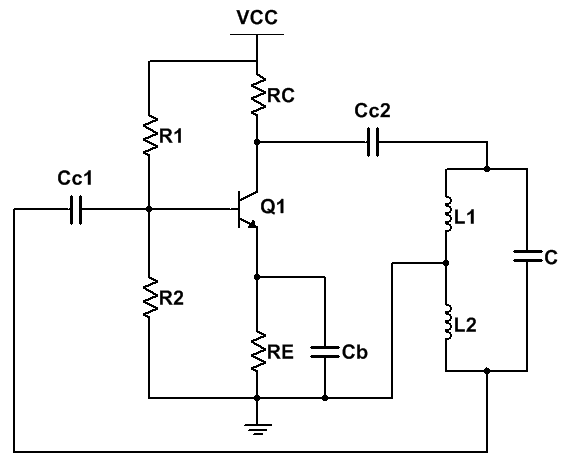# Wein Bridge Oscillator Calculator

Inputs:
Calculate Fr & B from R & C:

Results:

Calculate R & B from Fr & C:

Results:The above online Wienbridge calculator helps you to calculate the resistor and capacitor values for desired resonant frequency.

### Equations Used:

$$F_{r}= \frac{1}{2\pi RC},$$ $$B=\frac{1}{\sqrt{9-(X_{c}/R-R/X_{c})^2}}$$

# RC Phase Shift Oscillator Calculator

Inputs:
Calculate Fr,Rf & ACL from R & C

Results:

Calculate R,Rf & ACL from Fr & C

V

Results:## About RC Phase Shift Oscillator Calculator

The above online RC phase shift oscillator calculator is free and calculates the resistor, capacitor, resonant frequency, feedback fraction for the oscillator circuit.

Tutorials on design of Colpitts oscillator are as follows:
(1) 2N2222 Phase Shift Oscillator (2) BC547 Transistor Phase Shift Oscillator

### Equations Used:

$$F_{r}= \frac{1}{2\pi RC\sqrt{6}},$$ $$R_{b}=29R,$$ $$A_{CL}=\frac{R_{f}}{R},$$ $$B=\frac{1}{29}$$

# Colpitts Oscillator Calculator

Inputs:
Calculate Fr,B & ACL(min) from L, C1 & C2

Results:Calculate L,B & ACL(min) from Fr,C1 & C2

Results:

Calculate C2,B & ACL(min) given Fr,C1 & L

Results:

The above online Colpitts Oscillator calculator is free and calculates the resistor, capacitor, resonant frequency, feedback fraction for the oscillator circuit.

Tutorials on design of Colpitts oscillator are as follows:
(1) Colpitts oscillator with LM358 and TL072 Op-Amps

Equations Used:
$$F_{r}= \frac{1}{2\pi \sqrt{LC}},$$ $$C=\frac{C_{1}C_{2}}{C_{1}+C_{2}},$$ $$B=\frac{C_{1}}{C_{2}}$$

# Hartley Oscillator Calculator

Inputs:
Calculate Fr,B & ACL(min) from C, L1 & L2

Results:Calculate C,B & ACL(min) from Fr,L1 & L2

Results:

$$F_{r}=\frac{1}{2\pi \sqrt{LC}},$$ $$L=L_{1}+L_{2},$$ $$B=\frac{L_{2}}{L_{1}},$$ $$A_{CL(min)}=\frac{L_{1}}{L_{2}}$$